# CSE 1341 Sorting Searching Searching Sorting Searching data

• Slides: 23CSE 1341 Sorting & Searching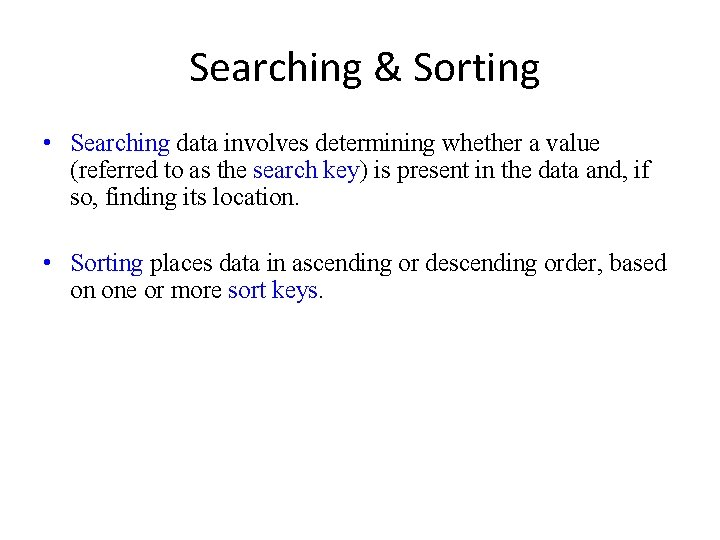Searching & Sorting • Searching data involves determining whether a value (referred to as the search key) is present in the data and, if so, finding its location. • Sorting places data in ascending or descending order, based on one or more sort keys.Linear Search • The linear search algorithm searches each element in an array sequentially. – If the search key does not match an element in the array, the algorithm tests each element, and when the end of the array is reached, informs the user that the search key is not present. – If the search key is in the array, the algorithm tests each element until it finds one that matches the search key and returns the index of that element. • If there are duplicate values in the array, linear search returns the index of the first element in the array that matches the search key.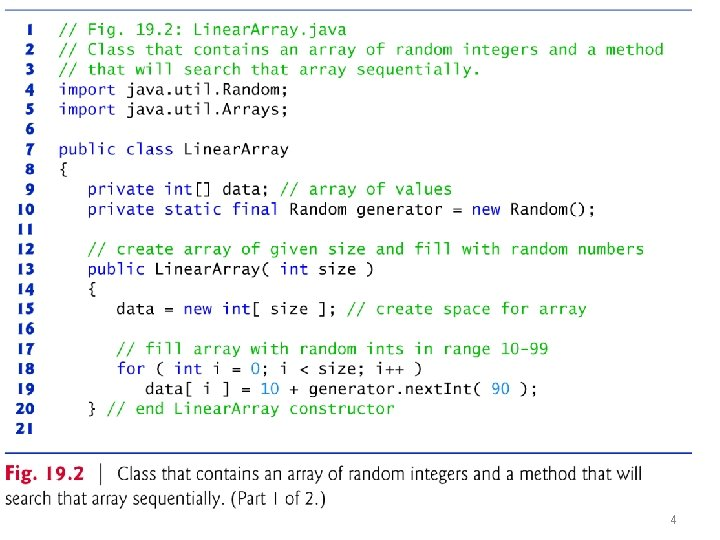4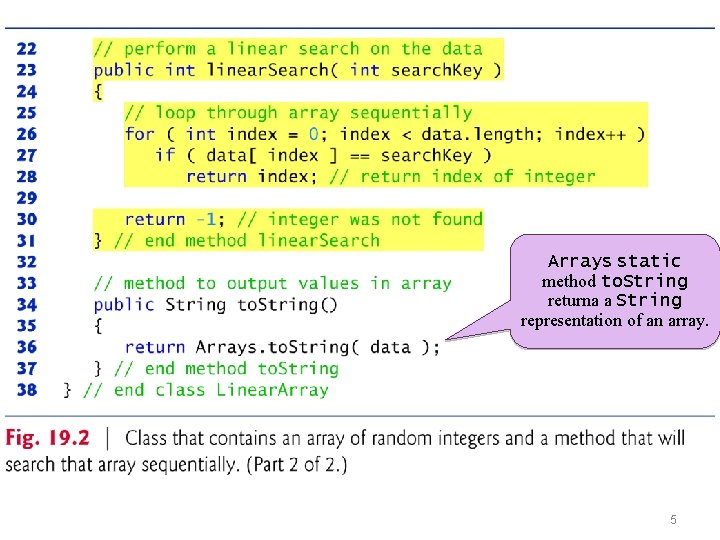Arrays static method to. String returna a String representation of an array. 5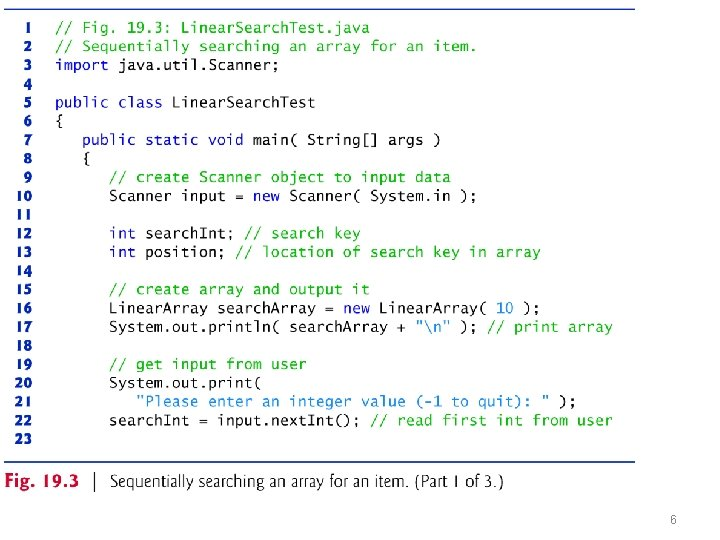678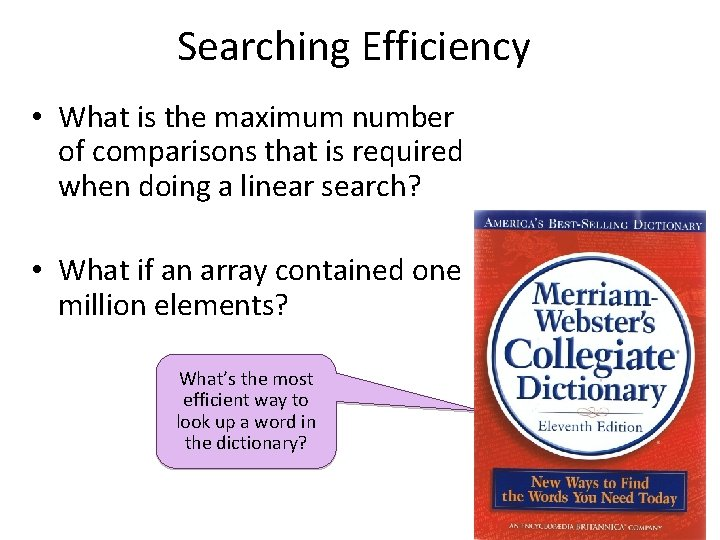Searching Efficiency • What is the maximum number of comparisons that is required when doing a linear search? • What if an array contained one million elements? What’s the most efficient way to look up a word in the dictionary?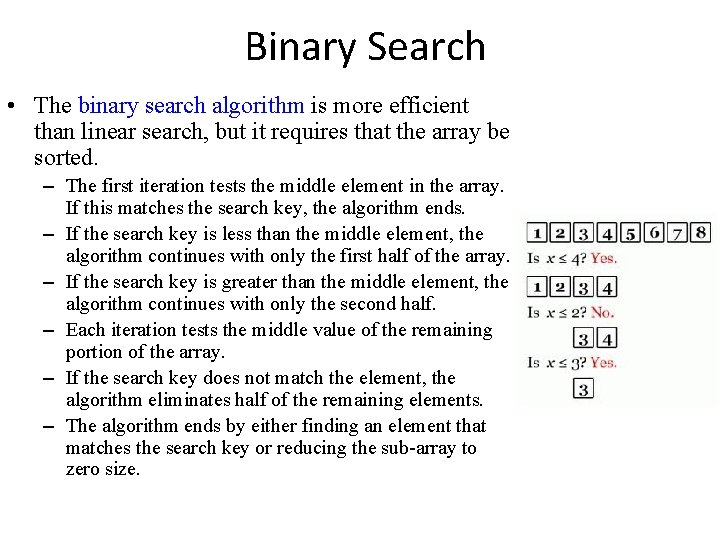Binary Search • The binary search algorithm is more efficient than linear search, but it requires that the array be sorted. – The first iteration tests the middle element in the array. If this matches the search key, the algorithm ends. – If the search key is less than the middle element, the algorithm continues with only the first half of the array. – If the search key is greater than the middle element, the algorithm continues with only the second half. – Each iteration tests the middle value of the remaining portion of the array. – If the search key does not match the element, the algorithm eliminates half of the remaining elements. – The algorithm ends by either finding an element that matches the search key or reducing the sub-array to zero size.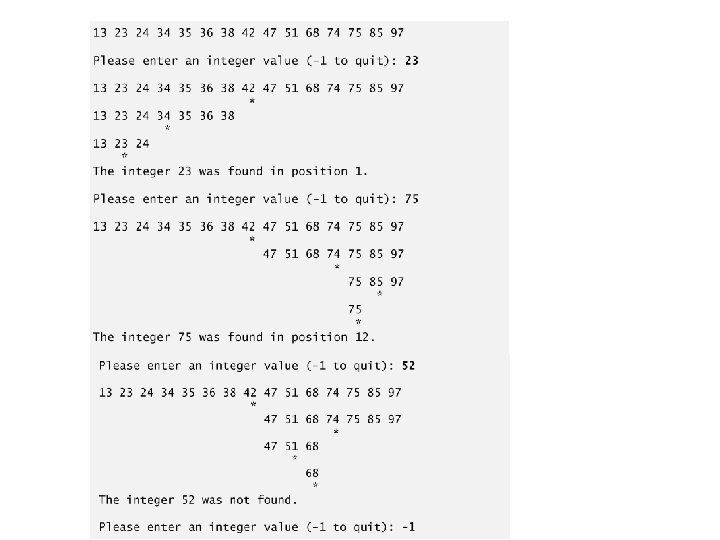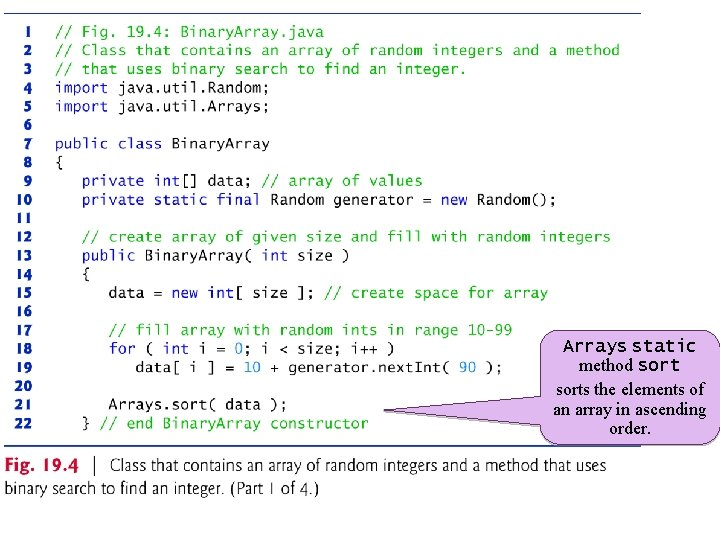Arrays static method sorts the elements of an array in ascending order.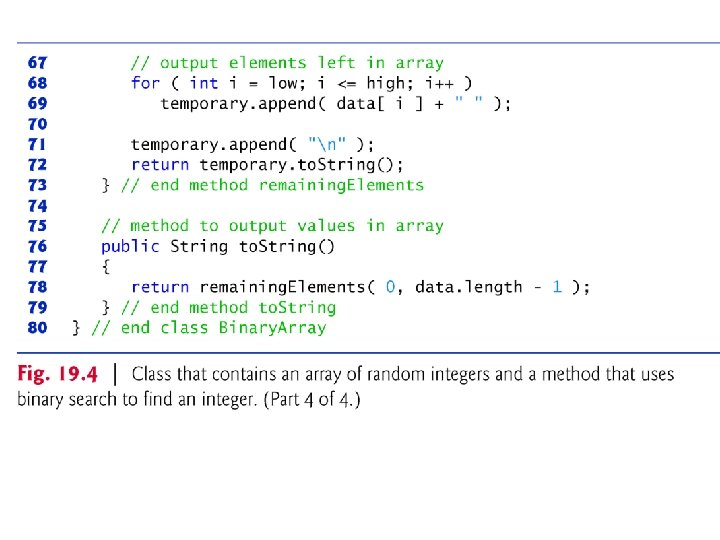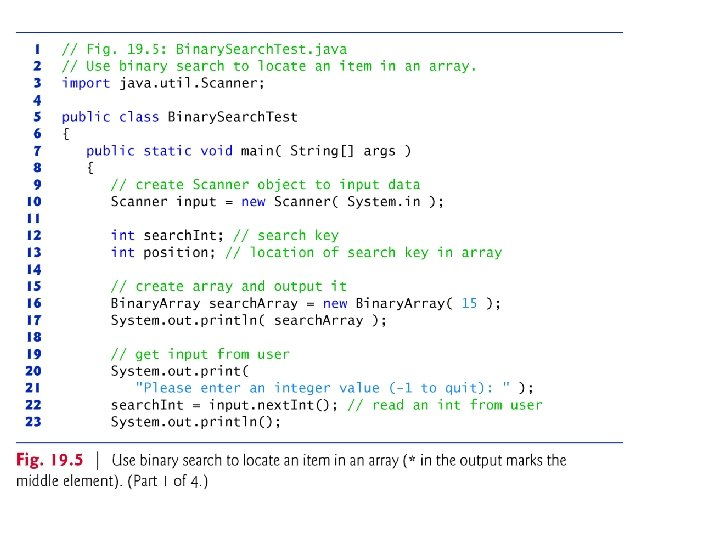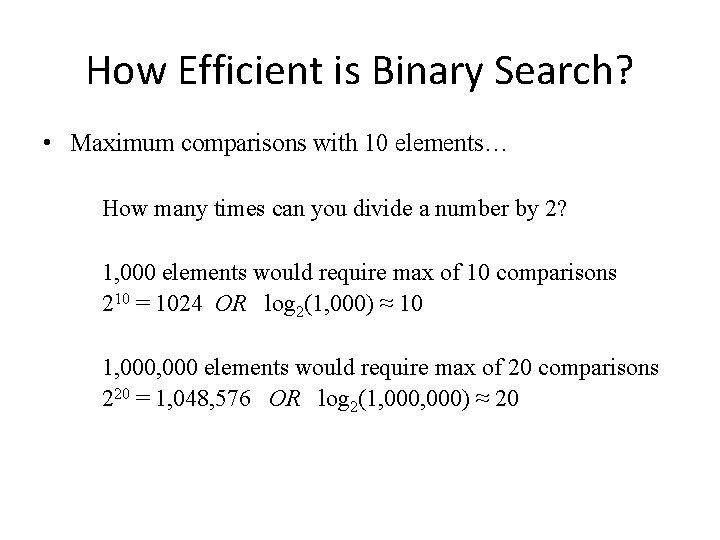How Efficient is Binary Search? • Maximum comparisons with 10 elements… How many times can you divide a number by 2? 1, 000 elements would require max of 10 comparisons 210 = 1024 OR log 2(1, 000) ≈ 10 1, 000 elements would require max of 20 comparisons 220 = 1, 048, 576 OR log 2(1, 000) ≈ 20Sorting Algorithms • Sorting data (i. e. , placing the data into some particular order, such as ascending or descending) is one of the most important computing applications. • An important item to understand about sorting is that the end result—the sorted array—will be the same no matter which algorithm you use to sort the array. • The choice of algorithm affects only the run time and memory use of the program.Selection Sort Find the smallest value and swap places with the first element Repeat for 2 -n, 3 -n, 4 -n, …. n-n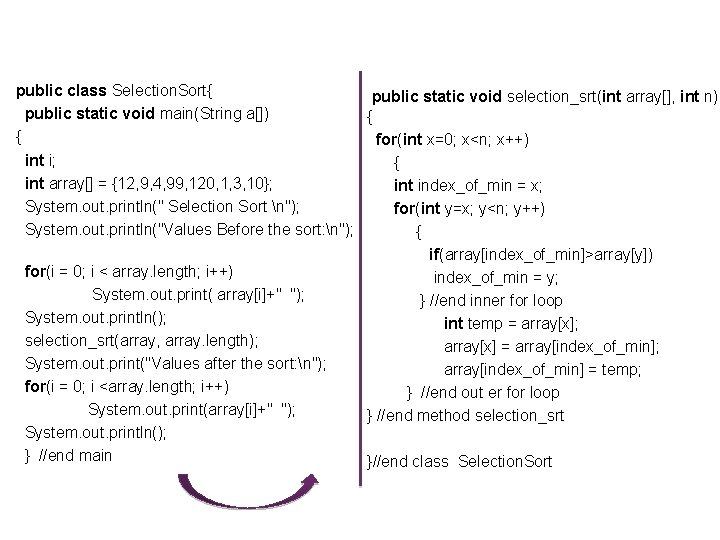public class Selection. Sort{ public static void selection_srt(int array[], int n) public static void main(String a[]) { { for(int x=0; x<n; x++) int i; { int array[] = {12, 9, 4, 99, 120, 1, 3, 10}; int index_of_min = x; System. out. println(" Selection Sort n"); for(int y=x; y<n; y++) System. out. println("Values Before the sort: n"); { if(array[index_of_min]>array[y]) for(i = 0; i < array. length; i++) index_of_min = y; System. out. print( array[i]+" "); } //end inner for loop System. out. println(); int temp = array[x]; selection_srt(array, array. length); array[x] = array[index_of_min]; System. out. print("Values after the sort: n"); array[index_of_min] = temp; for(i = 0; i <array. length; i++) } //end out er for loop System. out. print(array[i]+" "); } //end method selection_srt System. out. println(); } //end main }//end class Selection. SortBubble Sort Repeat n-1 timespublic class Bubble. Sort { public static void bubble_srt( int a[], int n ) public static void main(String a[]){ { int i; int i, j, t=0; int array[] = {12, 9, 4, 99, 120, 1, 3, 10}; for(i = 0; i < n; i++) System. out. println("Values Before the sort: n"); { for(i = 0; i < array. length; i++) for(j = 1; j < (n-i); j++) System. out. print( array[i]+" "); { System. out. println(); if(a[j-1] > a[j]) { t = a[j-1]; bubble_srt(array, array. length); a[j-1]=a[j]; a[j]=t; System. out. print("Values after the sort: n"); } //end if } //end inner for loop for(i = 0; i <array. length; i++) } //end outer for loop System. out. print(array[i]+" "); } //end method bubble_srt System. out. println(); }//end class Bubble. Sort }